### 在GPU上運行，性能是NumPy的11倍，這個Python庫你值得擁有Theano是Python的一個庫，為開源項目，在2008年，由Yoshua Bengio領導的加拿大蒙特利爾理工學院LISA實驗室開發。對於解決大量數據的問題，使用Theano可能獲得與手工用C實現差不多的性能。另外通過利用GPU，它能獲得比CPU上快很多數量級的性能。

• 如何安裝Theano。

• 符號變量是什麼。

• 如何設計符號計算圖。

• 函數的功能。

• 共享變量的妙用。

Theano開發者在2010年公佈的測試報吿中指出：在CPU上執行程序時，Theano程序性能是NumPy的1.8倍，而在GPU上是NumPy的11倍。這還是2010年的測試結果，近些年無論是Theano還是GPU，性能都有顯著提高。01 安裝

Theano支持CPU、GPU，如果使用GPU還需要安裝其驅動程序如CUDA等，限於篇幅，這裏只介紹CPU的，有關GPU的安裝，大家可參考：

http://www.deeplearning.net/software/theano/install.html

1. 安裝anaconda

anaconda官網：

``bash Anaconda3-4.3.1-Linux-x86_64.sh``

``conda list``

2. 安裝theano

``conda install theano``

3. 測試

``\$ PythonPython 3.6.0 |Anaconda custom (64-bit)| (default, Dec 23 2016, 12:22:00) [GCC 4.4.7 20120313 (Red Hat 4.4.7-1)] on linuxType "help", "copyright", "credits" or "license" for more information.>>> import theano>>>``

02 符號變量

``#導入需要的庫或模塊import theanofrom theano import tensor as T#初始化張量x=T.scalar(name='input',dtype='float32')w=T.scalar(name='weight',dtype='float32')b=T.scalar(name='bias',dtype='float32')z=w*x+b#編譯程序net_input=theano.function(inputs=[w,x,b],outputs=z)#執行程序print('net_input: %2f'% net_input(2.0,3.0,0.5))``

``net_input: 6.500000``

1. 使用內置的變量類型創建

``import theanofrom theano import tensor as Tx=T.scalar(name='input',dtype='float32')data=T.vector(name='data',dtype='float64')``

2. 自定義變量類型

``import theanofrom theano import tensor as Tmytype=T.TensorType('float64',broadcastable=(),name=None,sparse_grad=False)``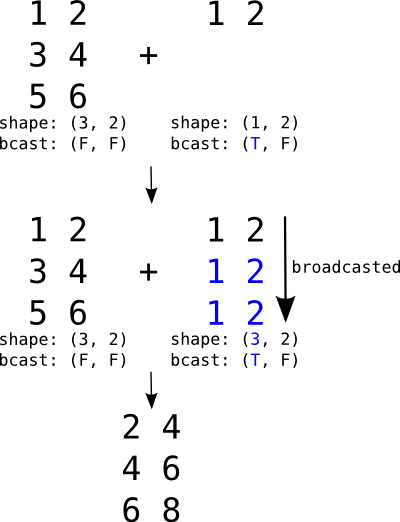▲圖2-1 廣播機制

``import theanoimport numpy as npimport theano.tensor as Tr = T.row()r.broadcastable# (True, False)mtr = T.matrix()mtr.broadcastable# (False, False)f_row = theano.function([r, mtr], [r + mtr])R = np.arange(1,3).reshape(1,2)print(R)#array([[1, 2]])M = np.arange(1,7).reshape(3, 2)print(M)#array([[1, 2],#       [3, 4],#       [5, 6]])f_row(R, M)#[array([[ 2.,  4.],#        [ 4.,  6.],#        [ 6.,  8.]])]``

3. 將Python類型變量或者NumPy類型變量轉化為Theano共享變量

``import theanoimport numpy as npimport theano.tensor as Tdata=np.array([[1,2],[3,4]])shared_data=theano.shared(data)type(shared_data)``

03 符號計算圖模型

Theano處理符號表達式時是通過把符號表達式轉換為一個計算圖（graph）來處理（TensorFlow也使用了這種方法，等到我們介紹TensorFlow時，大家可對比一下），符號計算圖的節點有：variable、type、apply和op。

• variable節點：即符號的變量節點，符號變量是符號表達式存放信息的數據結構，可以分為輸入符號和輸出符號。

• type節點：當定義了一種具體的變量類型以及變量的數據類型時，Theano為其指定數據存儲的限制條件。

• apply節點：把某一種類型的符號操作符應用到具體的符號變量中，與variable不同，apply節點無須由用户指定，一個apply節點包括3個字段：op、inputs、outputs。

• op節點：即操作符節點，定義了一種符號變量間的運算，如+、-、sum()、tanh()等。

Theano是將符號表達式的計算表示成計算圖。這些計算圖是由Apply 和 Variable將節點連接而組成，它們分別與函數的應用和數據相連接。操作由op 實例表示，而數據類型由type 實例表示。

``import theanoimport numpy as npimport theano.tensor as Tx = T.dmatrix('x')  y = T.dmatrix('y')  z = x + y  ``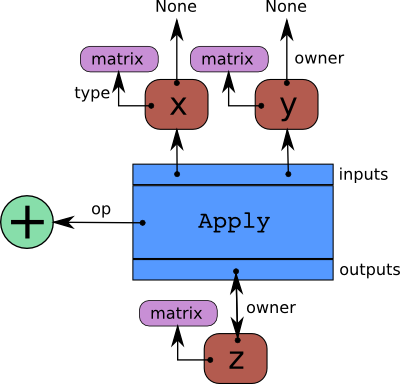▲圖2-2 符號計算圖

x和y的owner域的指向都是None，這是因為它們不是另一個計算的結果。如果它們中的一個變量是另一個計算的結果，那麼owner域將會指向另一個藍色盒。

04 函數

1. 函數定義的格式

``theano.function(inputs, outputs, mode=None, updates=None, givens=None, no_default_updates=False, accept_inplace=False, name=None,rebuild_strict=True, allow_input_downcast=None, profile=None, on_unused_input='raise')``

``import theano  x, y =theano.tensor.fscalars('x', 'y')  z1= x + y  z2=x*y  #定義x、y為自變量，z1、z2為函數返回值（因變量）f =theano.function([x,y],[z1,z2])  #返回當x=2，y=3的時候，函數f的因變量z1，z2的值print(f(2,3))``

``[array(5.0, dtype=float32), array(6.0, dtype=float32)]``

2. 自動求導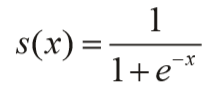``import theano  x =theano.tensor.fscalar('x')#定義一個float類型的變量x  y= 1 / (1 + theano.tensor.exp(-x))#定義變量y  dx=theano.grad(y,x)#偏導數函數  f= theano.function([x],dx)#定義函數f，輸入為x，輸出為s函數的偏導數  print(f(3))#計算當x=3的時候，函數y的偏導數``

``0.045176658779382706``

3. 更新共享變量參數

``import theanow= theano.shared(1)#定義一個共享變量w，其初始值為1  x=theano.tensor.iscalar('x')  f=theano.function([x], w, updates=[[w, w+x]])#定義函數自變量為x，因變量為w，當函數執行完畢後，更新參數w=w+x  print(f(3))#函數輸出為w  print(w.get_value())#這個時候可以看到w=w+x為4``

``1、4``

``import numpy  as npimport theano  import theano.tensor as T  rng = np.random  # 我們為了測試，自己生成10個樣本，每個樣本是3維的向量，然後用於訓練 N = 10feats = 3D = (rng.randn(N, feats).astype(np.float32), rng.randint(size=N, low=0, high=2).astype(np.float32))# 聲明自變量x、以及每個樣本對應的標籤y(訓練標籤)  x = T.matrix("x")y = T.vector("y")#隨機初始化參數w、b=0，為共享變量  w = theano.shared(rng.randn(feats), name="w")  b = theano.shared(0., name="b")#構造代價函數p_1 = 1 / (1 + T.exp(-T.dot(x, w) - b))   # s激活函數  xent = -y * T.log(p_1) - (1-y) * T.log(1-p_1) # 交叉商代價函數cost = xent.mean() + 0.01 * (w ** 2).sum()# 代價函數的平均值+L2正則項以防過擬合，其中權重衰減係數為0.01  gw, gb = T.grad(cost, [w, b])             #對總代價函數求參數的偏導數  prediction = p_1 > 0.5                    # 大於0.5預測值為1，否則為0.train = theano.function(inputs=[x,y],outputs=[prediction, xent],updates=((w, w - 0.1 * gw), (b, b - 0.1 * gb)))#訓練所需函數predict = theano.function(inputs=[x], outputs=prediction)#測試階段函數  #訓練  training_steps = 1000  for i in range(training_steps):      pred, err = train(D, D)      print (err.mean())#查看代價函數下降變化過程  ``

05 條件與循環

1. 條件判斷

Theano是一種符號語言，條件判斷不能直接使用Python的if語句。在Theano可以用ifelse和switch來表示判定語句。這兩個判定語句有何區別呢？

switch對每個輸出變量進行操作，ifelse只對一個滿足條件的變量操作。比如對語句：

``switch(cond, ift, iff) ``

``if cond then ift else iff``

ifelse只執行ift或者只執行iff。

``from theano import tensor as T  from theano.ifelse import ifelse  import theano,time,numpy  a,b=T.scalars('a','b')  x,y=T.matrices('x','y')  z_switch=T.switch(T.lt(a,b),T.mean(x),T.mean(y))#lt:a < b?  z_lazy=ifelse(T.lt(a,b),T.mean(x),T.mean(y))  #optimizer:optimizer的類型結構（可以簡化計算，增加計算的穩定性）  #linker:決定使用哪種方式進行編譯(C/Python) f_switch = theano.function([a, b, x, y], z_switch,mode=theano.Mode(linker='vm'))  f_lazyifelse = theano.function([a, b, x, y], z_lazy,mode=theano.Mode(linker='vm'))  val1 = 0.  val2 = 1.  big_mat1 = numpy.ones((1000, 100))  big_mat2 = numpy.ones((1000, 100))  n_times = 10  tic = time.clock()  for i in range(n_times):      f_switch(val1, val2, big_mat1, big_mat2)  print('time spent evaluating both values %f sec' % (time.clock() - tic))  tic = time.clock()  for i in range(n_times):      f_lazyifelse(val1, val2, big_mat1, big_mat2)  print('time spent evaluating one value %f sec' % (time.clock() - tic))  ``

``time spent evaluating both values 0.005268 sectime spent evaluating one value 0.007501 sec ``

2. 循環語句

scan是Theano中構建循環Graph的方法，scan是個靈活複雜的函數，任何用循環、遞歸或者跟序列有關的計算，都可以用scan完成。其格式如下：

``theano.scan(fn, sequences=None, outputs_info=None, non_sequences=None, n_steps=None, truncate_gradient=-1, go_backwards=False, mode=None, name=None, profile=False, allow_gc=None, strict=False)``

• sequences：scan進行迭代的變量，scan會在T.arange()生成的list上遍歷，例如下面的polynomial 例子。

• outputs_info：初始化fn的輸出變量，和輸出的shape一致。如果初始化值設為None，表示這個變量不需要初始值。

• non_sequences：fn函數用到的其他變量，迭代過程中不可改變（unchange）。

• n_steps：fn的迭代次數。

``import theanoimport theano.tensor as Timport numpy as np# 定義單步的函數,實現a*x^n# 輸入參數的順序要與下面scan的輸入參數對應def one_step(coef, power, x):    return coef * x ** powercoefs = T.ivector()  # 每步變化的值,係數組成的向量powers = T.ivector() # 每步變化的值,指數組成的向量x = T.iscalar()      # 每步不變的值,自變量# seq,out_info,non_seq與one_step函數的參數順序一一對應# 返回的result是每一項的符號表達式組成的listresult, updates = theano.scan(fn = one_step,                       sequences = [coefs, powers],                       outputs_info = None,                       non_sequences = x)# 每一項的值與輸入的函數關係f_poly = theano.function([x, coefs, powers], result, allow_input_downcast=True)coef_val = np.array([2,3,4,6,5])power_val = np.array([0,1,2,3,4])x_val = 10print("多項式各項的值: ",f_poly(x_val, coef_val, power_val))#scan返回的result是每一項的值，並沒有求和，如果我們只想要多項式的值，可以把f_poly寫成這樣：# 多項式每一項的和與輸入的函數關係f_poly = theano.function([x, coefs, powers], result.sum(), allow_input_downcast=True)print("多項式和的值：",f_poly(x_val, coef_val, power_val))``

``多項式各項的值:  [ 2   30   400  6000 50000]多項式和的值： 56432``

06 共享變量

``import theanoimport theano.tensor as Tfrom theano import sharedimport numpy as np#定義一個共享變量，並初始化為0state = shared(0)inc = T.iscalar('inc')accumulator = theano.function([inc], state, updates=[(state, state+inc)])# 打印state的初始值print(state.get_value())accumulator(1) # 進行一次函數調用# 函數返回後，state的值發生了變化print(state.get_value()) ``

07 小結

Theano基於NumPy，但性能方面又高於NumPy。因Theano採用了張量(Tensor)這個核心元素，在計算方面採用符號計算模型，而且採用共享變量、自動求導、利用GPU等適合於大數據、深度學習的方法，其他很多開發項目也深受這些技術和框架影響。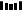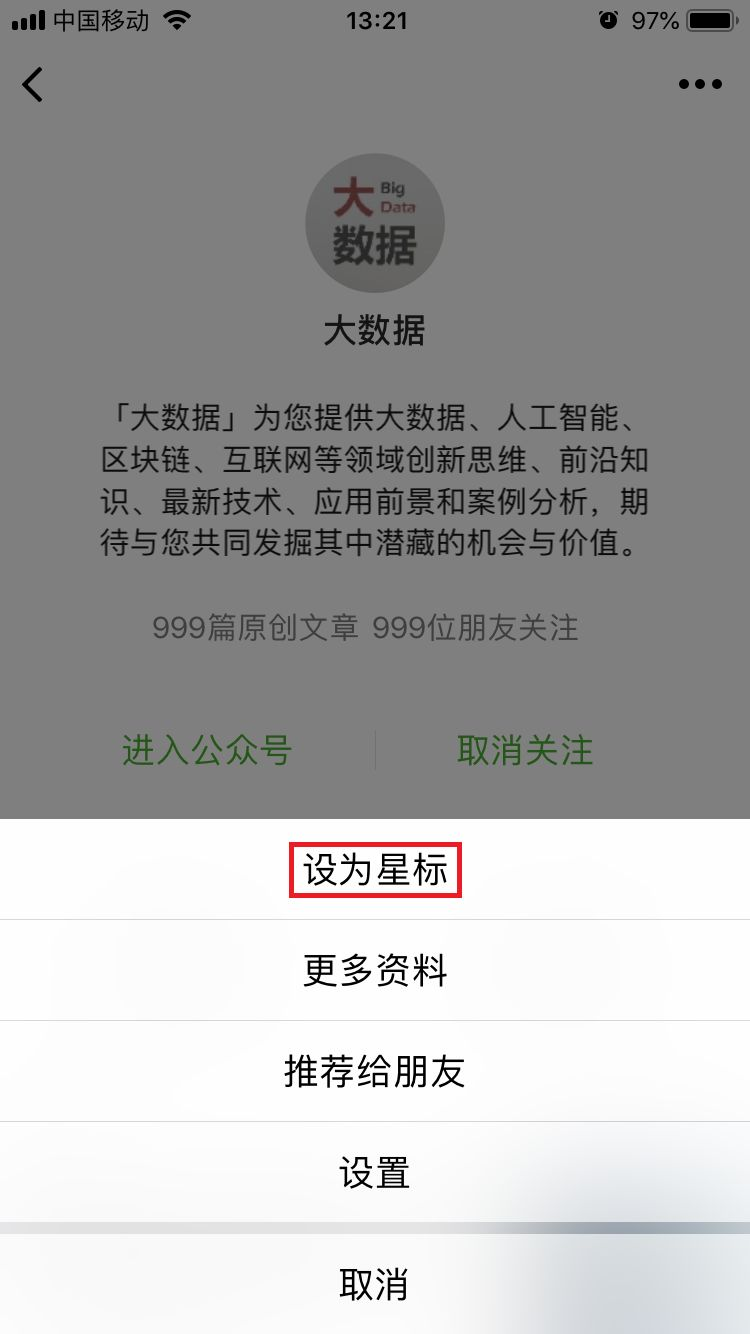PPT | 報吿 | 讀書 | 書單 | 乾貨

AI | 1024 | 段子 | 區塊鏈 | 數學

• 壓力、焦慮遠遠超出全國平均值，近4成程序員心理不健康？

• 從Python安裝到語法基礎，這才是小白都能懂的爬蟲教程

• 「π」裏藏着所有人的銀行卡密碼和生日？

• 什麼樣的數據才有價值？應該怎樣收集和處理？終於有人講明白了

Q: 關於Theano，你還有哪些經驗和技巧？點擊閲讀原文，瞭解更多